Latest Banking jobs   »   NIACL AO 2021 Result Out, Mains Exam Date For 300 AO Recruitment   »   Quantitative Aptitude Quiz For IBPS Clerk/NIACL...

# Quantitative Aptitude Quiz For IBPS Clerk/NIACL AO Prelims 2021- 23rd September

Directions (1-5): The data in the table given below shows the selling price, profit obtained and discount percentage on 4 items of a store. Some data are missing in this table and you have to calculate missing data according to the questions.
Study the data carefully and answer the following questions.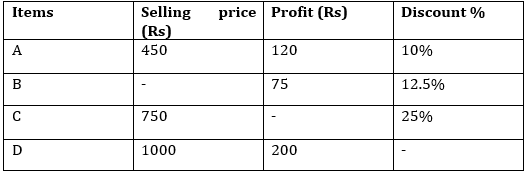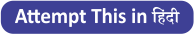Q1. What is the marked price of the article A?
(a) Rs 540
(b) Rs 460
(c) Rs 500
(d) Rs 600
(e) Rs 480

Q2. What is the selling price of the article B, if marked price of article B is 20% above the cost price?
(a) Rs 1775
(b) Rs 1500
(c) Rs 1850
(d) Rs 1625
(e) Rs 1575

Q3. If ratio between profit earned on article B and article C is 3 : 4, find the cost price of article C?
(a) Rs 650
(b) Rs 600
(c) Rs 680
(d) Rs 700
(e) Rs 600

Q4. Find the profit percentage earned on article D?
(a) 20%
(b) 22.5%
(c) 15%
(d) 25%
(e) 17.5%

Q5. If profit amount on article B and discount amount of article B is same, then find the selling price of article B?
(a) Rs 450
(b) Rs 525
(c) Rs 625
(d) Rs 575
(e) Rs 475

Directions (6-10): The table given below shows the no. of books published by 4 different publishers in 4 months. Study the data and answer the following questions.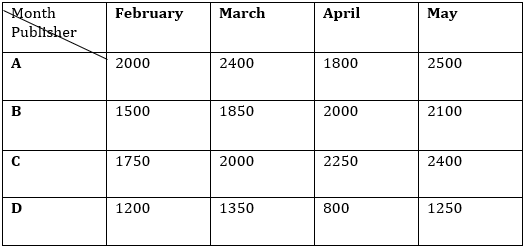Q6. What is the average no. of books published by A in all the given months?
(a) 1740
(b) 2275
(c) 2050
(d) 2175
(e) 2250

Q7. Books published by B in February and March together is what percent more/less than that by C in March and April? (approximate)
(a) 21%
(b) 24%
(c) 16%
(d) 12%
(e) 27%

Q8. Find the ratio between books published by C to D in all given months.
(a) 23 : 45
(b) 24 : 43
(c) 42 : 23
(d) 41 : 25
(e) 23 : 42

Q9. Find the revenue obtained by B in March is how much more/less than that by D in same month, if selling price of book is Rs 120 and all books are sold. (Note – cost price and selling price of each book is same for all publishers)
(a) Rs 50,000
(b) Rs 40,000
(c) Rs 55,000
(d) Rs 70,000
(e) Rs 60,000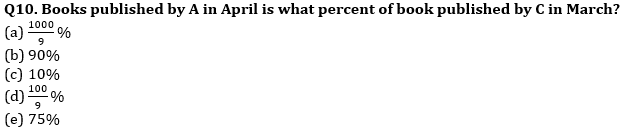Directions (11-15): Pie chart given below shows the percentage distribution of marks obtained in History out of total marks obtained by five students (i.e. Deepak, Hemant, Manoj, Vikash and Shivam) of a class in History. There are only three subjects in class i.e. (Hindi, English and History).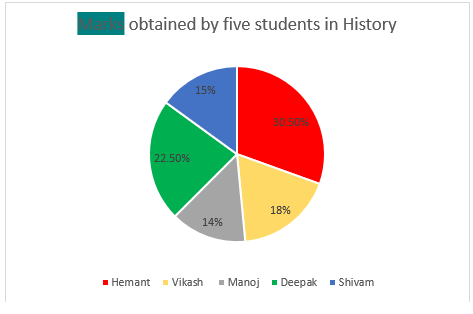Q11. If marks obtained by Hemant in history is 82.5 more than marks obtained by Manoj in same subject. Find total marks obtained by Hemant, Deepak and Manoj in History?
(a) 315
(b) 305
(c) 325
(d) 335
(e) 275

Q12. Manoj’s score in Hindi and English is 33⅓% more and 10 more than that of Vikash and Shivam scored in History respectively and total score of Manoj in three subjects is 275, find marks scored by Deepak in History?
(a) 100
(b) 105
(c) 112.5
(d) 75.5
(e) 97.5

Q13. What is the ratio of marks obtained by Deepak to Vikash and Manoj together in History out of total marks obtained by five students in History?
(a) 45:64
(b) 45:61
(c) 61:64
(d) 15:19
(e) 15:17

Q14. Marks obtained by Deepak in History out of total marks obtained by five students in History is 135, find marks obtained by Manoj in History?
(a) 112
(b) 84
(c) 98
(d) 70
(e) None of these.

Q15. If total marks obtained by all 5 students in History is 600. Find marks obtained by Hemant is how much more/less than that of Deepak in History.
(a) 36
(b) 28
(c) 54
(d) 48
(e) 44

Solutions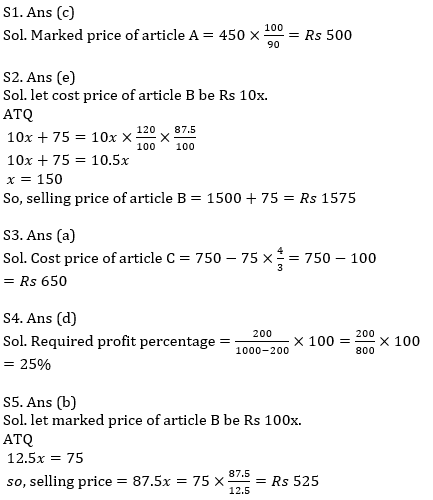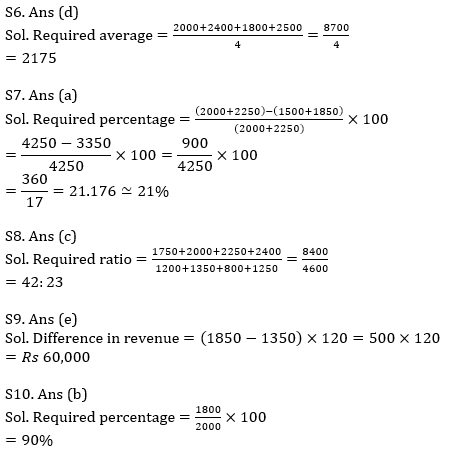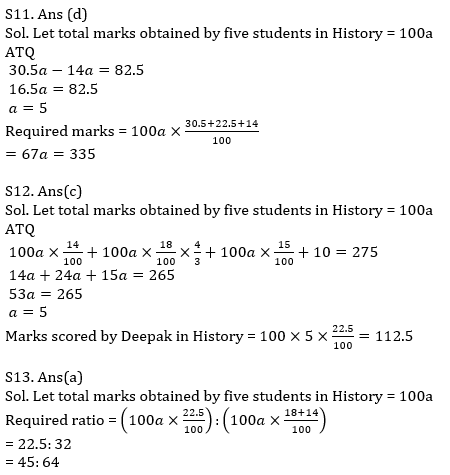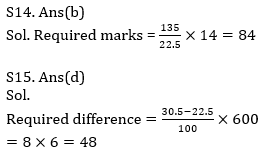Click Here to Register for Bank Exams 2021 Preparation Material#### Congratulations!Download Hindu Review of October 2021: Free PDF